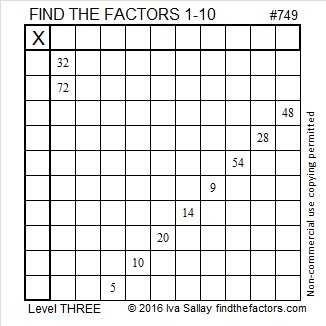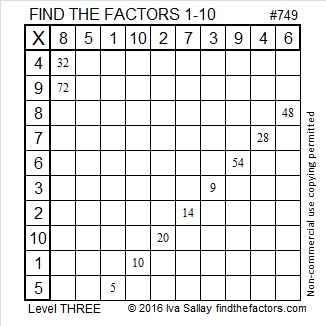# 749 and Level 3

Obviously 749 can be evenly divided by 7.

• 749 is a composite number.
• Prime factorization: 749 = 7 x 107
• The exponents in the prime factorization are 1 and 1. Adding one to each and multiplying we get (1 + 1)(1 + 1) = 2 x 2 = 4. Therefore 749 has exactly 4 factors.
• Factors of 749: 1, 7, 107, 749
• Factor pairs: 749 = 1 x 749 or 7 x 107
• 749 has no square factors that allow its square root to be simplified. √749 ≈ 27.367864.Here’s today’s puzzle:Print the puzzles or type the solution on this excel file: 10 Factors 2016-01-18

—————————————

Here’s a little more about the number 749:

749 can be written as the sum of consecutive numbers three different ways:

• 374 + 375 = 749; that’s 2 consecutive numbers.
• 104 + 105 + 106 + 107 + 108 + 109 + 110 = 749; that’s 7 consecutive numbers.
• 47 + 48 + 49 + 50 + 51 + 52 + 53 + 54 + 55 + 56 + 57 + 58 + 59 + 60 = 749; that’s 14 consecutive numbers.

749 is the sum of three consecutive prime numbers: 241 + 251 + 257 = 749.

749 is the sum of three cubes eight different ways:

1. 27² + 4² + 2² = 749
2. 26² + 8² + 3² = 749
3. 24² + 13² + 2² = 749
4. 22² + 16² + 3² = 749
5. 22² + 12² + 11² = 749
6. 20² + 18² + 5² = 749
7. 19² + 18² + 8² = 749
8. 18² + 16² + 13² = 749

749 is a palindrome in two different bases:

• 525 BASE 12; note that 5(144) + 2(12) + 5(1) = 749
• 1C1 BASE 22 (C = 12 base 10); note that 1(22²) + 12(22) + 1(1) = 749

—————————————

A Logical Approach to solve a FIND THE FACTORS puzzle: Find the column or row with two clues and find their common factor. (None of the factors are greater than 10.)  Write the corresponding factors in the factor column (1st column) and factor row (top row).  Because this is a level three puzzle, you have now written a factor at the top of the factor column. Continue to work from the top of the factor column to the bottom, finding factors and filling in the factor column and the factor row one cell at a time as you go.This site uses Akismet to reduce spam. Learn how your comment data is processed.